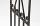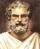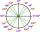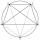Circumferential angle

Vertices of the triangle ΔABC lies on circle and divided it into arcs in the ratio 2:2:9.

Determine the size of the angles of the triangle ΔABC.

Result

A =  27.69 °
B =  27.69 °
C =  124.62 °

Solution:Leave us a comment of example and its solution (i.e. if it is still somewhat unclear...):Be the first to comment!To solve this example are needed these knowledge from mathematics:

Need help calculate sum, simplify or multiply fractions? Try our fraction calculator. See also our trigonometric triangle calculator.

Next similar examples:

1. Inscribed triangleTo a circle is inscribed triangle so that the it's vertexes divide circle into 3 arcs. The length of the arcs are in the ratio 2:3:7. Determine the interior angles of a triangle.
2. Ruler and compassUse a ruler and compass to construct a triangle ABC with AB 5cm BAC 60° and ACB 45°.
3. SemicircleIn the semicircle with center S and the diameter AB is constructed equilateral triangle SBC. What is the magnitude of the angle ∠SAC?
4. ConstructConstruct a triangle ABC inscribed circle has a radius r = 2 cm, the angle alpha = 50 degrees = 8 cm. Make a sketch, analysis, construction and description.
5. Clock faceclock face is given. Numbers 10 and 5, and 3 and 8 are connected by straight lines. Calculate the size of their angles.
6. AnglesIn the triangle ABC, the ratio of angles is: a:b = 4: 5. The angle c is 36°. How big are the angles a, b?
7. Angles in triangleThe triangle is ratio of the angles β:γ = 6:8. Angle α is 40° greater than β. What are the size of angles of the triangle?
8. Internal anglesFind the internal angles of the triangle ABC if the angle at the vertex C is twice the angle at the B and the angle at the vertex B is 4 degrees smaller than the angle at the vertex A.
9. A triangleA triangle has an angle that is 63.1 other 2 are in ratio of 2:5 What are the measurements of the two angles?
10. Triangle SSAConstruct a triangle ABC if |AB| = 5cm va = 3cm, CAB = 50 °. It is to create the analysis and construction steps.
11. Triangle 42Triangle BCA. Angles A=119° B=(3y+14) C=4y. What is measure of triangle BCA=?
12. Reference angleFind the reference angle of each angle:What is greater angle? -0.7 radians or -0.7π radians?It is true that the middle traverse bisects the triangle?The median of the triangle LMN is away from vertex N 84 cm. Calculate the length of the median, which start at N.How to divide the circle into 10 parts (geometrically)?We want to prove the sentence: If the natural number n is divisible by six, then n is divisible by three. From what assumption we started?